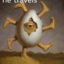MathematicsOpenStudy (anonymous):

solve. X+10/ x squared -2 = 4/xOpenStudy (anonymous):

$\frac{x+10}{x^2}-2=\frac{4}{x}$?OpenStudy (anonymous):

noOpenStudy (anonymous):

x squared -2 is the denominatorOpenStudy (anonymous):

or $\frac{x+10}{x^2-2}=\frac{4}{x}$OpenStudy (anonymous):

cross multiply and solve the resulting quadratic equation. first step is $x(x+10)=4(x^2-2)$OpenStudy (anonymous):

yes this oneOpenStudy (anonymous):

$x^2+10x=4x^2-8$ $3x^2-10x-8=0$ and if you are lucky it factors and if not use the formulaOpenStudy (anonymous):

okOpenStudy (anonymous):

$(x-4)(3x+2)=0$ etc

Latest QuestionsShelbiLW: A 3.0 m uniform beam of mass 15 kg is pivoted 1.0 m from the end as shown below. A 35 kg child sits 0.
5 hours ago 0 Replies 0 Medalsryleighmullins22: Answer the question below using the following amortization table. Month Principal Paid Interest Paid Balance 21 \$654.
5 hours ago 0 Replies 0 MedalsTonycoolkid21: help
5 hours ago 8 Replies 0 MedalsTonycoolkid21: help
5 hours ago 15 Replies 3 MedalsRx1nyDxyz: Help If the supply of a product increases, then the price decreases*** the price
7 hours ago 21 Replies 1 Medalshototodorokii: (not from a test/quiz)Nomenclature is governed by certain universal rules. Which
7 hours ago 0 Replies 0 Medalscrispyrat: A guide to sqrt and cbrt
6 hours ago 21 Replies 3 MedalsxXQuintonXx: help please(ss below)
8 hours ago 5 Replies 1 Medalajfknkjfa: Prove that the two circles shown below are similar. (10 points) Circle C is shown with a center at negative 3, 1 and a radius of 4.
7 hours ago 3 Replies 1 Medalrxcklesskaisher: idk wdh dis is
8 hours ago 2 Replies 0 Medals